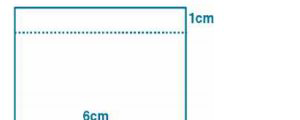# ML Aggarwal Solutions Class 9 Mathematics Solutions for Mensuration Exercise 16.4 in Chapter 16 - Mensuration

A rectangular container, whose base is a square of side 6 cm, stands on a horizontal table and holds

water up to 1 cm from the top. When a cube is placed in the water and is completely submerged, the water

rises to the top and 2 \mathrm{cm}^{3} of water overflows, calculate the volume of the cube.

If the base of the rectangular container is a square

l = 6 cm and b = 6 cm

When a cube is placed in it, the water rises to the top i.e. through height 1 cm and 2 \mathrm{cm}^{3}We know that

Volume of cube = Volume of water displaced

Substituting the values ML Aggarwal Solutions for Class 9 Maths Chapter 16 -

Mensuration

= 6 × 6 × 1 + 2

= 36 + 2

=38 \mathrm{cm}^{3}

Related Questions

Lido

Courses

Teachers

Book a Demo with us

Syllabus

Maths
CBSE
Maths
ICSE
Science
CBSE

Science
ICSE
English
CBSE
English
ICSE
Coding

Terms & Policies

Selina Question Bank

Maths
Physics
Biology

Allied Question Bank

Chemistry
Connect with us on social media!